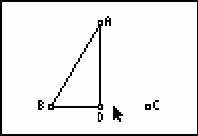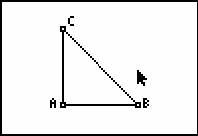# Activities

•##### Download

•• ##### Subject Area

• Math: Geometry: Triangles

• ##### Author9-12

50 Minutes

• ##### Device
• TI-83 Plus Family
• TI-84 Plus
• TI-84 Plus Silver Edition
•TI-84 Plus C Silver Edition
•TI-84 Plus CE
• ##### Software

TI Connect™
TI Connect™ CE

• ##### Accessories

TI Connectivity Cable
Unit-to-Unit link cables

• ##### Report an Issue

Special Triangles#### Activity Overview

Students visualize the construction of special right triangles and find the ratios of the sides.

#### Key Steps

•Students use Cabri™ Jr. to investigate special right triangles and their trigonometric ratios.

Students begin by constructing an isosceles triangle. Then they construct a perpendicular from the vertex opposite the base to the midpoint of the base. This forms two right triangles with angle measure 30, 60, and 90 degrees.

•Students will hide one equilateral triangle and measure the lengths of each leg and the hypotenuse. They use the Calculate tool to find the ratios BD:AB, AD:AB, and AD:BD. As students then drag point C, they should see that the ratios remain constant. Students should also observe that the ratio of lengths of the longer side to that of the shorter side is 1.73, or the square root of three.